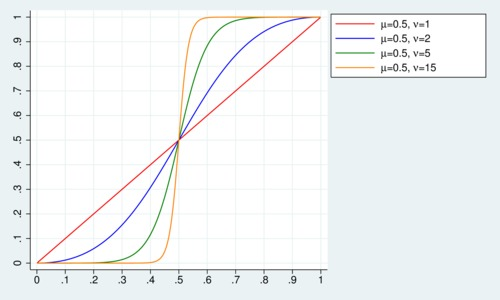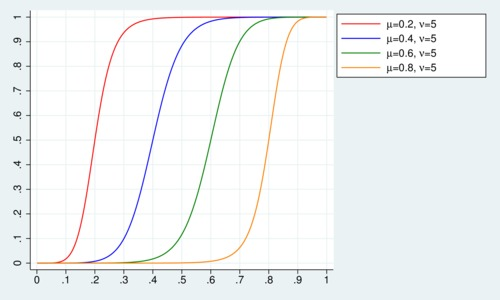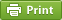Werner's Blog — Opinion, Analysis, Commentary
A sigmoid-logit probability function for the (0,1) domain

In certain economic applications we deal with distributions on a [0,1] scale. For example, we may want to model a [0,1] attribute of individuals, where we use a [0,1] scale to divide the population into a continuous range. Sometime one can use a uniform distribution for this purpose and let the attribute vary from [0,1] along with the population share. Another common approach divides the [0,1] range into two groups, a "zero"-attribute group with a $$0<\alpha<1$$ share of the population and a "one"-attribute group with a $$1-\alpha$$ share of the population, so that the mean is $$1-\alpha$$. This is a step function in terms of density.

Once in a while it can be advantageous to model a population in the [0,1] range with an attribute that clusters closely around one point $$\mu\in(0,1)$$ so that most people have a similar propensity for a particular attribute, and only few have a propensity that is close to zero or one. There is one useful logistic function that captures this type of behaviour. The usefulness of this function depends on the fact that the location parameter defines the median, and the shape parameter defines how compact the density is.

There are a number of statistical distribution function that cover the (0,1)→(0,1) domain, fo rexample the Beta function, or the logit-normal distribution The downside is that these probability functions start looking a bit odd for the problem at hand of modeling a narrow distribution about one point of the (0,1) domain.

Consider the following logistic cumulative distribution function (CDF) that approximates a step function with a location parameter $$0<\mu<1$$ at which the distribution function equals 1/2, and a shape parameter $$\nu≥1$$ that captures the steepness of the step function: $\Phi(x;\mu,\nu)=\left[1+\left(\frac{x\cdot(1-\mu)}{\mu\cdot(1-x)}\right)^{-\nu}\right]^{-1}$ For $$\mu=0.5$$ and $$\nu=1$$ the distribution function is simply the 45-degree line. As $$\nu$$ increases, the distribution function increasingly becomes a step function. The first chart shows what $$\Phi(x)$$ looks like for different values of $$\nu$$, all centered around $$\mu=0.5$$.The next chart shows what the distribution function looks like if the shape parameter is held constant at $$\nu=5$$ and the location parameter $$\mu$$ is varied.The median of the above distribution is always equal to $$\mu$$. What can be said about the mean and variance of this distribution? The probability density function (PDF) is $\phi(x;\mu,\nu) =\frac{\nu}{x(1-x)}\cdot\frac{Z(x;\mu,\nu)}{(1+Z(x;\mu,\nu))^2}$ with $Z(x;\mu,\nu)\equiv\left[\frac{x\cdot(1-\mu)}{\mu\cdot(1-x)}\right]^{-\nu}$ The probability density function reaches a peak of $$\nu$$ exactly at $$\mu$$. The mean of the distribution is 0.5 when the $$\mu=0.5$$. As $$\nu\rightarrow\infty$$ gets large, the mean of the distribution approaches $$\mu$$. For practical purposes, when $$\nu>10$$, this is numerically a very tight approximation. When $$\nu$$ is close to one, however, there is a small deviation and the mean is slightly larger than the median when $$\mu<0.5$$, and slightly smaller than the median when $$\mu>0.5$$. These deviations are still sufficiently small so that the statement "the mean is approximately $$\mu$$" remains valid.

I have not yet found any specific reference to this distribution function in the literature, although this does not mean it hasn't already been named somewhere by someone. If a statistician can tell me the name for this function, I would be obliged. Does this CDF has a name yet? If not, I propose the name sigmoid-logit distribution function because it uses the logit function to map $$x$$ to the infinite real domain, and the sigmoid function (the inverse logit function) to map the transformed result back to the (0,1) domain.

Posted on Saturday, November 3, 2018 at 15:30 — #EconometricsRecent Blog Entries

Topics

Months

Subscribe to RSS feed© 2023  Prof. Werner Antweiler, University of British Columbia.